1. 向量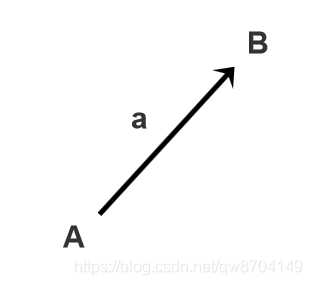A 点指向 B 点的一个有向线段，称为向量 a

a = B - A 用有向线段的结束点B减去起始点A就得到这个向量 a

|a| 表示向量a的长度

â 表示单位向量，â = a / |a|

2. 向量的矩阵表示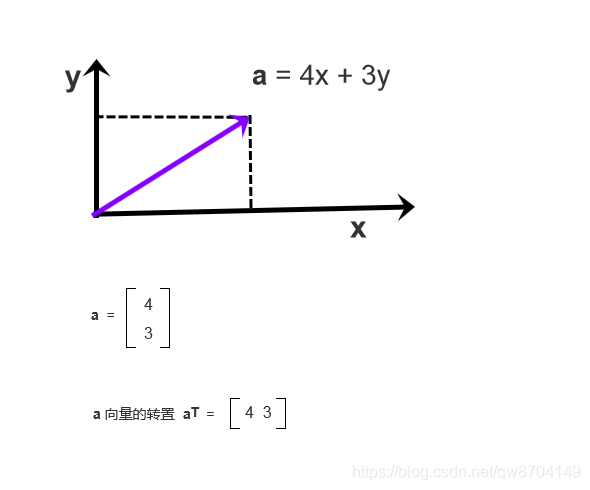3. 向量加法

3.1. 三角形定则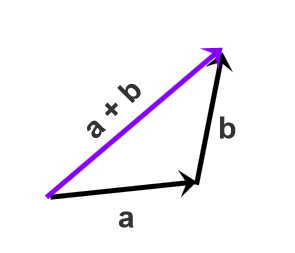3.2. 平行四边形定则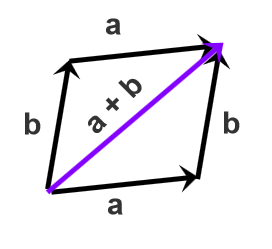4. 向量减法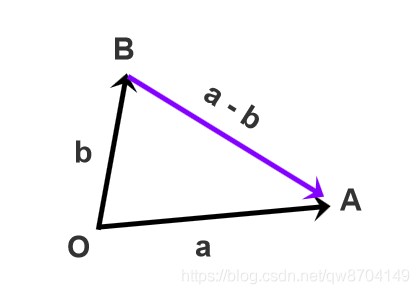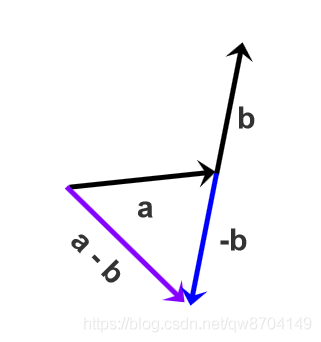5. 向量的点积（乘）

5.1. 点积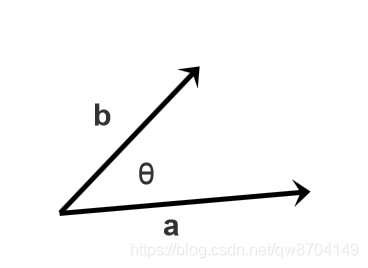$$\boldsymbol{a} \cdot \boldsymbol{b} = |\boldsymbol{a}| |\boldsymbol{b}| \boldsymbol{cos} \theta$$

$$\text{cos} \theta = \frac{\boldsymbol {a} \cdot \boldsymbol{b}} { |\boldsymbol{a}| |\boldsymbol{b}| }$$

$$\boldsymbol{cos} \theta = \hat{\boldsymbol{a}} \cdot \hat{\boldsymbol{b}}$$

5.2. 向量点乘的矩阵表示法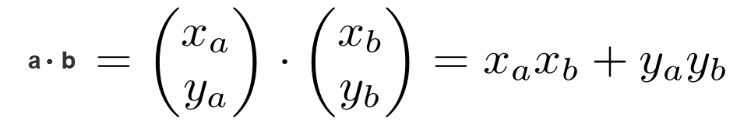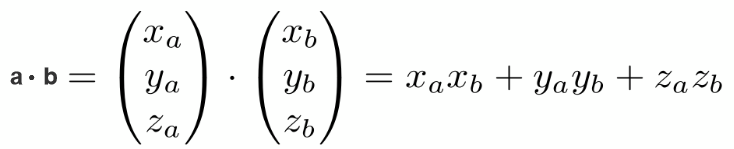5.3. 向量点乘适用的运算定律

 - 交换律 a · b = b · a

 - 分配律 a · (b + c) = a · b + a · c

 - 结合律 (ka) · b = a · (kb) = k(a · b)

5.4. 一个向量投影到另一个向量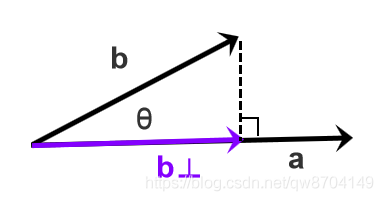$$\boldsymbol{b} ⊥ = k \hat{\boldsymbol{a}}$$

$$|\boldsymbol{b} ⊥| = |\boldsymbol{b}| \boldsymbol{cos} \theta$$

5.5. 向量点乘的应用

5.5.1. 计算两个向量的夹角

$$\text{cos} \theta = \frac{\boldsymbol {a} \cdot \boldsymbol{b}} { |\boldsymbol{a}| |\boldsymbol{b}| }$$

5.5.2. 判断两个向量的方向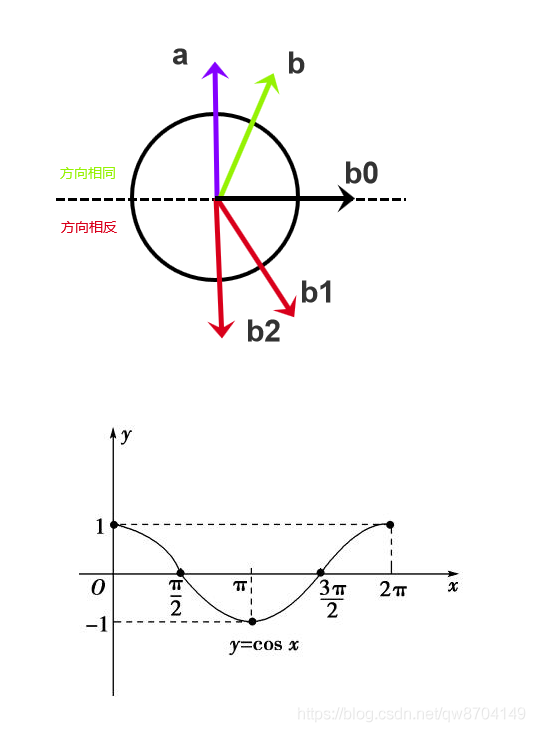$$\text{cos} \theta = \frac{\boldsymbol {a} \cdot \boldsymbol{b}} { |\boldsymbol{a}| |\boldsymbol{b}| }$$

$$\boldsymbol{a} \cdot \boldsymbol{b} = \boldsymbol{cos} \theta$$

6. 向量的叉积（乘）

6.1. 叉积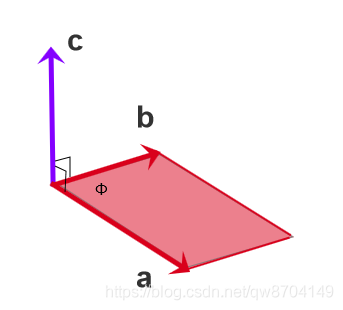6.3. 向量叉乘的一些性质

 - 向量 a 叉乘向量 b 的结果为向量 c. 向量 c 垂直于向量 a， 向量 c 垂直于向量 b，继而向量 c 垂直于向量 a与向量 b组成的平面

 - 向量 a 叉乘向量 b 等于负的向量 b 叉乘向量 aa x b = -b x a

 - 两个向量的叉乘的模长等于两个向量的模长相乘再乘以两个向量夹角的正弦值 |a x b| = |a| |b| sinΦ

 - 两个向量平行，因为sin0 = 0，则得到 |a x b| = 00 代表0向量. |a x b| 的值是以向量 a 和向量 b 为边组成的平行四边形的面积

6.4. 向量叉乘矩阵表示法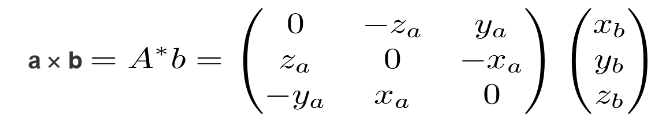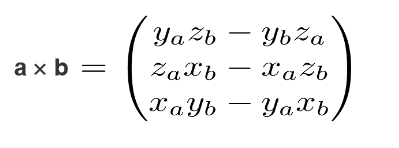6.5. 向量叉乘适用的运算定律

 - 分配律 a x (b + c) = a x b + a x c

 - 结合律 a x (kb) = k(a x b)

6.6. 向量叉乘的应用

6.6.1. 判断一个向量在另一个的左侧还是右侧

 - 向量 a 叉乘向量 b > 0, b 向量在 a 向量的左侧

 - 向量 a 叉乘向量 b < 0, b 向量在 a 向量的右侧

6.6.2. 判断一个点是否在三角形内部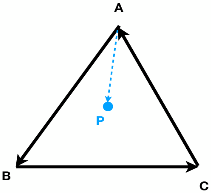- 把三角形的三条边按一定的顺序依次连接，组成三个向量，上图中是按逆时针方向连接

 - 取其中的一个向量AB，构造一个向量AP，从向量的起点A指向P点，向量AB叉乘向量AP，结果 > 0，所以 APAB 的左侧

 - 取下一个向量BC，计算向量BC叉乘向量BP，结果 > 0， 得到BP 也在 BC 的左侧

 - 取下一个向量CA，计算向量CA叉乘向量CP，结果 > 0， 得到CP 也在 CA 的左侧

 - 由第2,3,4步的结果推断出P点在三角形内部，如果第2,3,4步中有一个结果有一个不在左侧则P点不在三角形内部

Last modification：January 11th, 2022 at 11:49 pm